• Call Now

1800-102-2727•

## Ampere's Circuital Law - Magnetic field due to a cylindrical wire, solenoid, toroid, Modified form of Ampere’s law, Practice Problems, FAQs

Biot and Savart, proposed a law which would help us calculate the magnetic field due to a straight conductor or a circular loop of coil. On the other hand, the magnetic field produced in the vicinity of a straight wire carrying current can be calculated using Ampere’s circuital law. Ampere made use of an imaginary surface called the Amperian loop, which takes into account only the current enclosed in the loop, and not currents lying outside the loop. Using the law, magnetic fields created around coils like solenoid and toroid can be calculated as well. Ampere’s law is analogous to Gauss’ law–the latter takes into account the electric field created due to charges which are enclosed by an imaginary surface called Gaussian surface. In this article, we will explore Ampere’s circuital law in detail.

• Ampere’s Circuital Law derivation
• Magnetic field due to a cylindrical wire
• Magnetic field due to a solenoid
• Magnetic field due to a toroid
• Modified form of Ampere’s law
• Practice problems
• FAQs

## Ampere’s Circuital Law

Let us consider an imaginary surface called the Amperian loop as shown. $\stackrel{\to }{dl}$ is an infinitesimal element along the loop. The currents inside the loop are i1 and i2 as shown in the figure.

It states that the line integral of the quantity $\stackrel{\to }{B}.\stackrel{\to }{dl}$ is equal to 0 times the total current(ienclosed) enclosed inside the loop. Mathematically,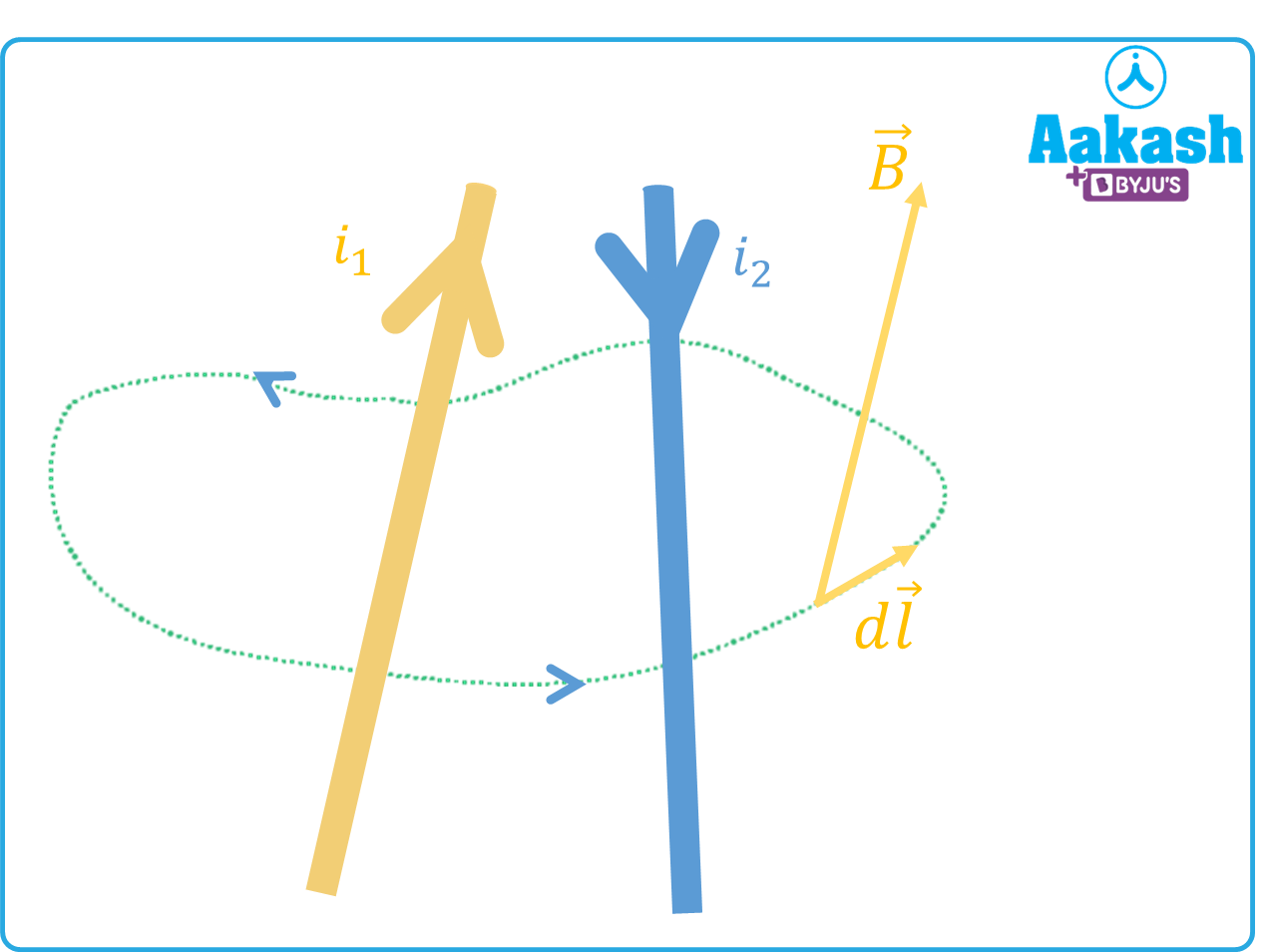${\oint }_{}^{}\stackrel{\to }{\mathbit{B}}.\stackrel{\to }{\mathbit{d}\mathbit{l}}={\mathbit{\mu }}_{0}{\mathbit{i}}_{\mathbit{t}\mathbit{o}\mathbit{t}\mathbit{a}\mathbit{l}}$

${\mu }_{0}=4\mathbit{\pi }×{10}^{-7}\frac{\mathbit{H}}{\mathbit{m}}$ is called the permeability of free space.

Applying Right hand thumb rule, the thumb points upward in the case of i1 and downwards in the case of i2. Therefore, the magnetic field (indicated by four fingers) due to i1 comes out of the plane of the paper and due to i2 would go into the plane of the paper.

${\mathbit{i}}_{\mathbit{t}\mathbit{o}\mathbit{t}\mathbit{a}\mathbit{l}}={\mathbit{i}}_{1}-{\mathbit{i}}_{2}$

## Magnetic field due to a cylindrical wire

Let us consider a solid cylinder of radius R which carries a current i which is uniformly distributed throughout the wire. We consider three points to calculate the magnetic field.

(i)Outside the cylinder(r>R)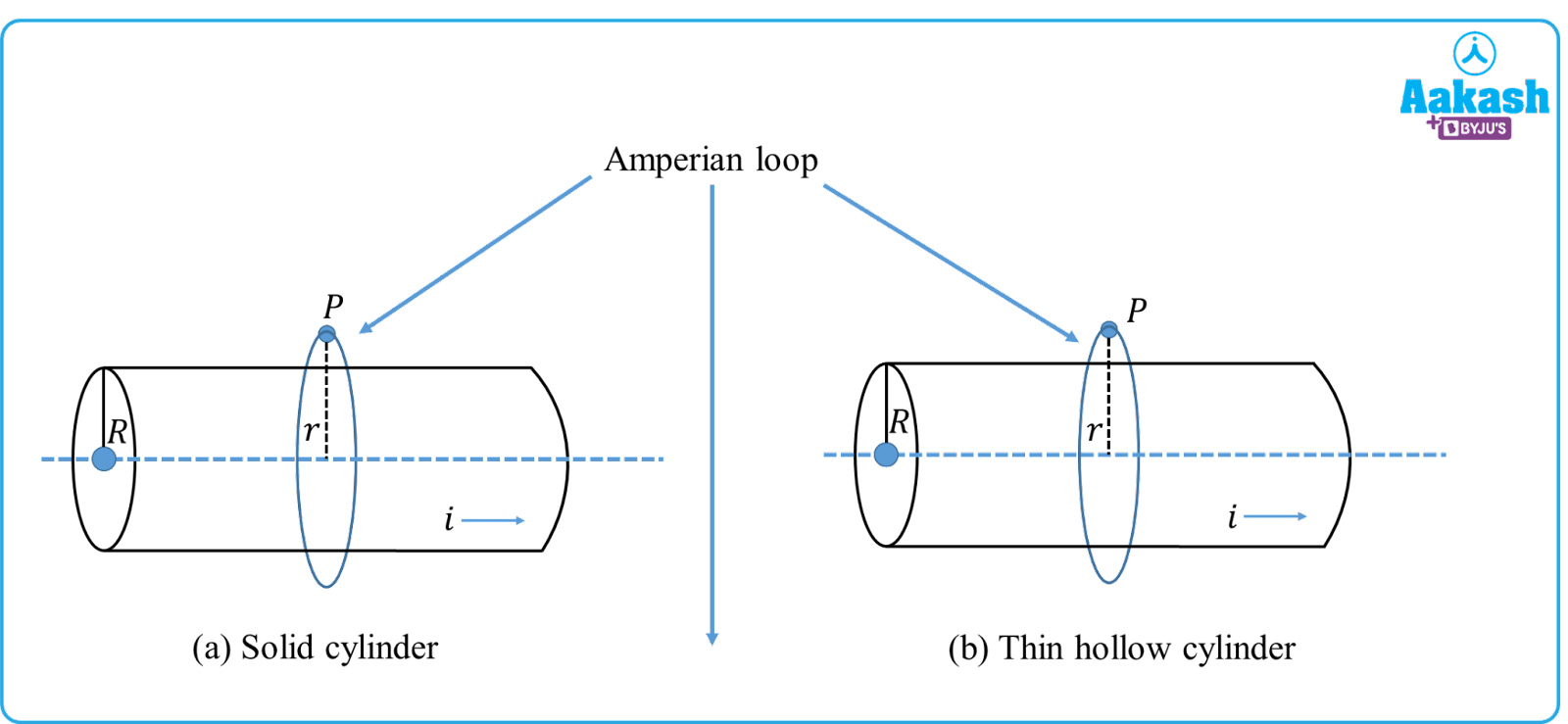Applying Ampere’s circuital law,

${\oint }_{}^{}{\stackrel{\to }{\mathbit{B}}}_{\mathbit{o}\mathbit{u}\mathbit{t}}.\stackrel{\to }{\mathbit{d}\mathbit{l}}={\mathbit{\mu }}_{0}{\mathbit{i}}_{\mathbit{t}\mathbit{o}\mathbit{t}\mathbit{a}\mathbit{l}}$

Since the point outside the cylinder, the net current enclosed by it would be i.e ${\mathbit{i}}_{\mathbit{t}\mathbit{o}\mathbit{t}\mathbit{a}\mathbit{l}}=\mathbit{i}$

The line integral of ${\stackrel{\to }{\mathbit{B}}}_{\mathbit{o}\mathbit{u}\mathbit{t}}{\oint }_{}^{}\stackrel{\to }{\mathbit{d}\mathbit{l}}={\mathbit{B}}_{\mathbit{o}\mathbit{u}\mathbit{t}}\left(2\mathbit{\pi }\mathbit{r}\right)$

${\mathbit{B}}_{\mathbit{o}\mathbit{u}\mathbit{t}}\left(2\mathbit{\pi }\mathbit{r}\right)={\mathbit{\mu }}_{0}\left(\mathbit{i}\right)$

${\mathbit{B}}_{\mathbit{o}\mathbit{u}\mathbit{t}}=\frac{{\mu }_{0}\mathbit{i}}{2\mathbit{\pi }\mathbit{r}}$

(ii)For a point inside the cylinder(r<R)

The point P shown lies inside the solid cylinder. Let the current enclosed by the Amperian loop be i'.${i}^{\text{'}}=i\frac{\pi {r}^{2}}{\pi {R}^{2}}{i}^{\text{'}}=i\frac{{r}^{2}}{{R}^{2}}$

${\oint }_{}^{}\stackrel{\to }{\mathbit{B}}.\stackrel{\to }{\mathbit{d}\mathbit{l}}={\mathbit{\mu }}_{0}{{\mathbit{i}}^{\mathbit{\text{'}}}}_{}$

${\mathbit{B}}_{\mathbit{i}\mathbit{n}}=\frac{{\mu }_{0}\mathbit{i}\mathbit{r}}{2\mathbit{\pi }{\mathbit{R}}^{2}}$

(iii)For a point lying on the surface of the cylinder, (r=R) , the current enclosed by the Amperian loop would be i.

${\mathbit{B}}_{\mathbit{s}\mathbit{u}\mathbit{r}\mathbit{f}\mathbit{a}\mathbit{c}\mathbit{e}}\left(2\mathbit{\pi }\mathbit{R}\right)={\mathbit{\mu }}_{0}\left(\mathbit{i}\right)$

${\mathbit{B}}_{\mathbit{s}\mathbit{u}\mathbit{r}\mathbit{f}\mathbit{a}\mathbit{c}\mathbit{e}}=\frac{{\mu }_{0}i}{2\pi R}$

The following graph shows the variation of magnetic field at different points on the cylindrical tube.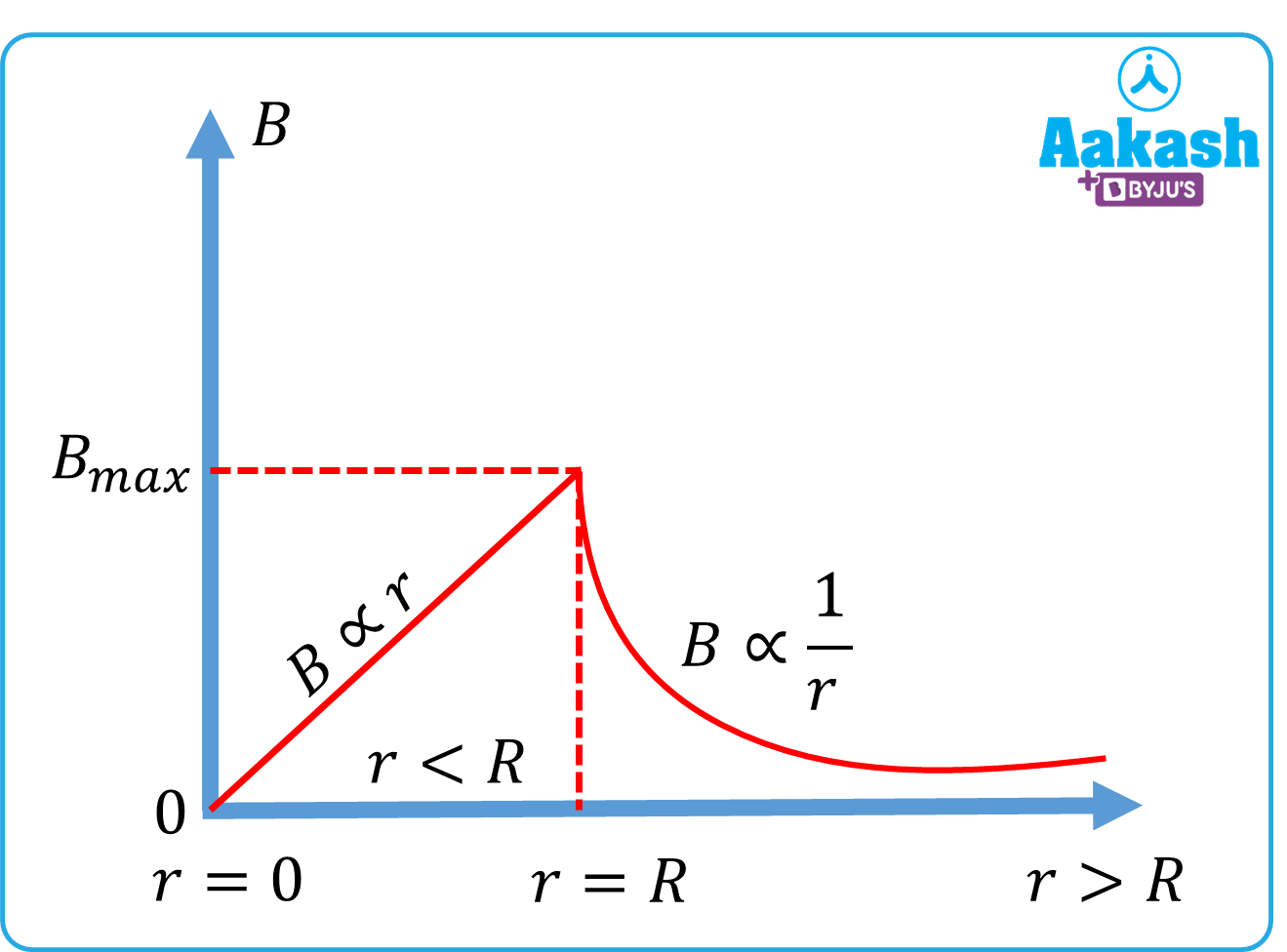## Magnetic field due to a solenoid

A solenoid generates a magnetic field when current is passed through it. Let us consider a solenoid having N turns and current i flowing through the windings.

To find the magnetic field on the axis of the solenoid, let us assume an Amperian loop of length l.

The magnetic field along different parts of the closed loop ABCDA can be calculated as,

${\oint }_{AB}^{}\stackrel{\to }{B}.\stackrel{\to }{dl}+{\oint }_{BC}^{}\stackrel{\to }{B}.\stackrel{\to }{dl}+{\oint }_{CD}^{}\stackrel{\to }{B}.\stackrel{\to }{dl+}{\oint }_{DA}^{}\stackrel{\to }{B}.\stackrel{\to }{dl}$

${\oint }_{AB}^{}\stackrel{\to }{B}.\stackrel{\to }{dl}=0$ as it lies outside the Amperian loop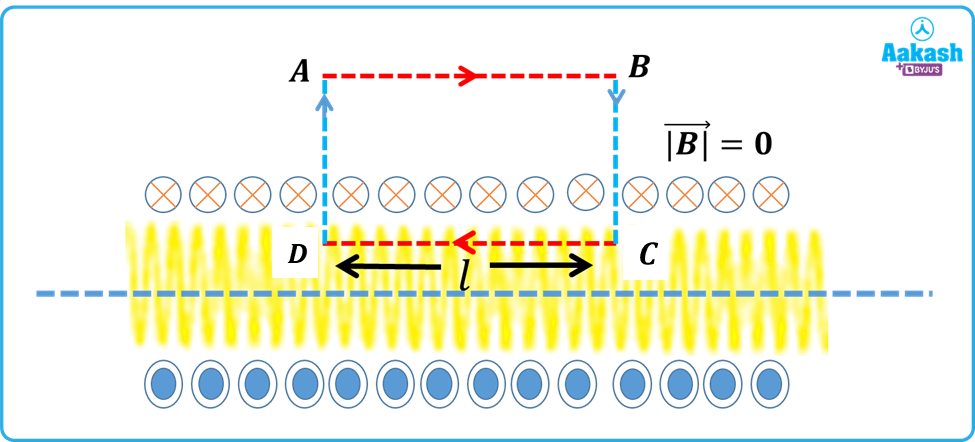The net magnetic field due to the solenoid,

Here ${i}_{total}=Ni$ is the total current enclosed by the solenoid.

N=n l, where n is the number of turns per unit length.

## Magnetic field due to a toroid

A toroid is a coil that is bent in the shape of a ring. Unlike a solenoid, the magnetic field produced in a toroid is not uniform. The earliest form of the toroid is called a Rowland Ring. It consists of a coil with many turns wound on a donut shaped ring.

Let us consider a toroid with inner radius r1 and outer radius r2. The current flowing in it is i.

1) For a point lying inside the toroid, (r<r1<r2)

Let Bin be the magnetic field inside the toroid. Then according to Ampere’s circuital law,

The current enclosed by the Amperian loop (denoted by yellow dotted lines) is zero.

Hence, the magnetic field inside the toroid is zero.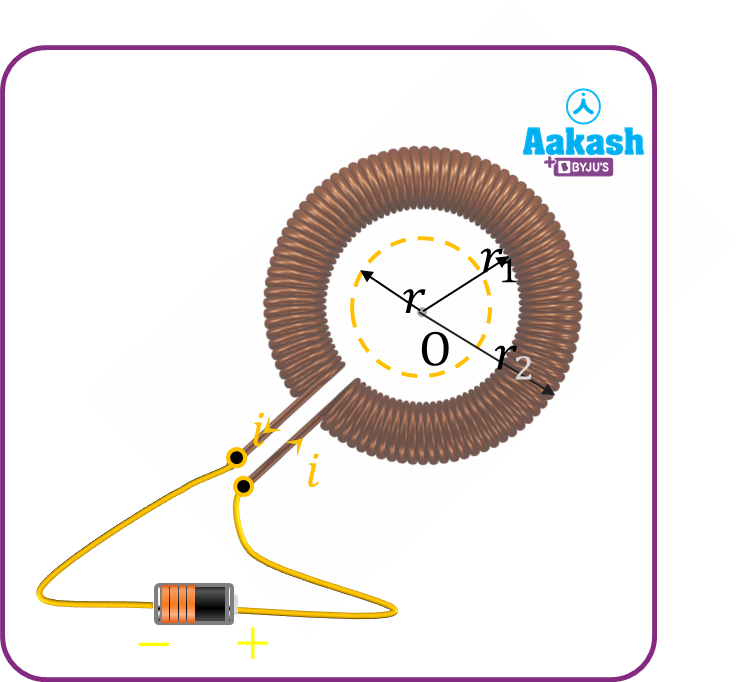2)For a point lying on the surface of the toroid, (r1<r<r2)

Let ${\stackrel{\to }{B}}_{surface}$ be the magnetic field on the windings of the toroid.

Here $n=\frac{N}{2\pi r}$ is the number of turns per unit length.

3) For a point lying outside the toroid, r>r2.

Applying Ampere’s circuital law,

The net current enclosed by the Amperian loop in this case is zero, hence ${i}_{total}=0.$.

So, ${B}_{out}=0.$.

Hence, the magnetic field outside the toroid is zero.

## Modified form of Ampere’s law

Ampere’s law failed to hold valid when a time varying current was involved. This is especially true in capacitors. Conduction current(ic) is responsible for charging the plates of a capacitor.

But the modified version introduced by Maxwell ( and hence came to known as Ampere-Maxwell law) can be written as

id- displacement current between the plates of a capacitor. It is a time varying current which generates a varying electric field.

Video explanation

From 52:17 to 1:10:16

## Practice problems

Q1.Using Ampere’s circuital law, find the magnetic field on the surface of a solid cylindrical tube whose radius is 10 cm and carries a uniform current of 1 A.

Given, current i= 1 A,

The magnitude of magnetic field on the surface of the cylindrical tube,

Q2.In the above question, calculate the magnetic field at a distance of 5 cm from the center of the tube.

Here, distance 5 cm lies inside the tube. Hence, r=5 cm=0.05 m

The magnetic field at a point inside the cylinder,

Q3.A toroid coil has a total of 300 windings. The radius of the coil is found to be 50 cm. Using Ampere’s circuital law, calculate the magnetic field at a point on the surface of the toroid.

Given, current flowing through the toroid is 2 A

No. of turns N=300 turns.

Using Ampere’s circuital law, the magnetic field at a point on the surface of the toroid

Q4.Using Ampere’s circuital law, calculate the magnetic field at a point outside a solid cylindrical tube whose radius is 20 cm and carries a uniform current of 2 A. The point lies at a distance of 30 cm from the center of the tube.

Here,the point is located at distance r= 0.3 m

Radius of the cylinder, R=20 cm=0.2 m

Current flowing, i=2 A

## FAQs

Q1.Give one limitation of Ampere’s law?
Ampere’s law is applicable only for steady currents. For time varying currents, they produce a time varying electric field, and Ampere’s law fails when electric field starts varying with time. This is why the modified version of Ampere’s law is used in capacitors.

Q2.What are applications of Ampere’s law?
Ampere’s law can be used to determine the magnetic field created due to current flowing in solenoids, toroids and straight conductors. Hence, it can be used to determine the strength of the magnetic field created by electromagnets and inductors.

Q3.What are the conditions for Ampere’s law to be valid?
For the law to be valid, we must consider the Amperian loop to be a smooth symmetrical path without any breaks in between. At all points on the loop, the magnitude of the magnetic field should be the same.Additionally, the current responsible for the magnetic field should be a steady current.

Q4.What is the modified form of Ampere’s law?
Maxwell modified Ampere’s law by introducing the concept of time varying electric field. A changing magnetic field induces a changing electric field and vice versa. This leads to the concept of displacement current–the time varying current between the plates of a capacitor.Talk to our expert
Resend OTP Timer =
By submitting up, I agree to receive all the Whatsapp communication on my registered number and Aakash terms and conditions and privacy policy